Скачать презентацию Title Density Equations Date Objective Practice density equations

6b683a267675fbdc703644b601dc5404.ppt

• Количество слайдов: 26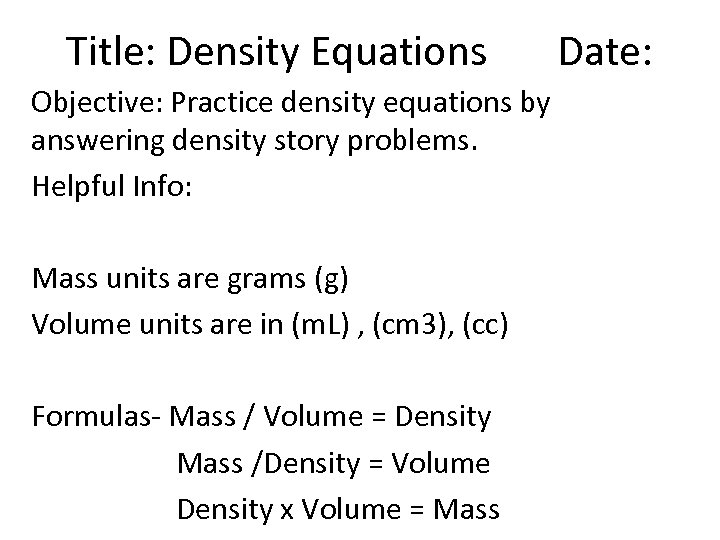Title: Density Equations Date: Objective: Practice density equations by answering density story problems. Helpful Info: Mass units are grams (g) Volume units are in (m. L) , (cm 3), (cc) Formulas- Mass / Volume = Density Mass /Density = Volume Density x Volume = Mass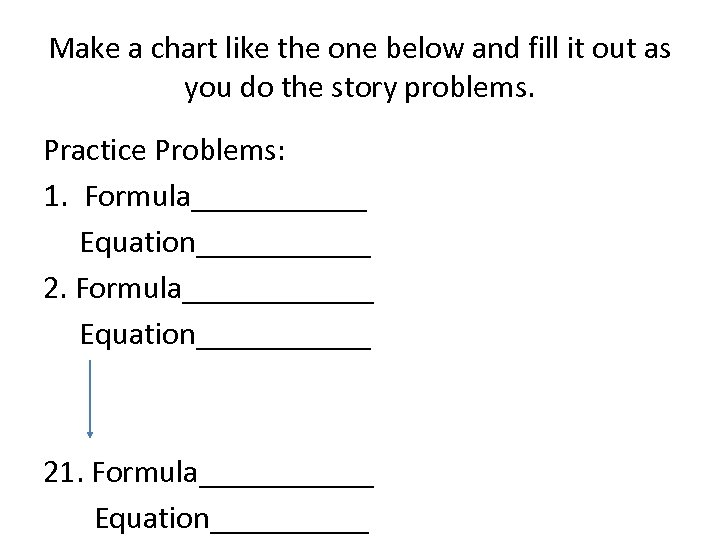Make a chart like the one below and fill it out as you do the story problems. Practice Problems: 1. Formula______ Equation______ 21. Formula______ Equation_____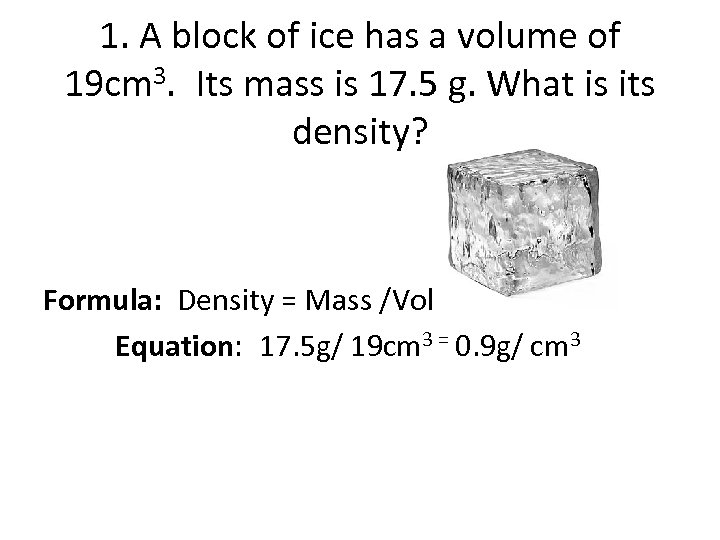1. A block of ice has a volume of 19 cm 3. Its mass is 17. 5 g. What is its density? Formula: Density = Mass /Volume Equation: 17. 5 g/ 19 cm 3 = 0. 9 g/ cm 3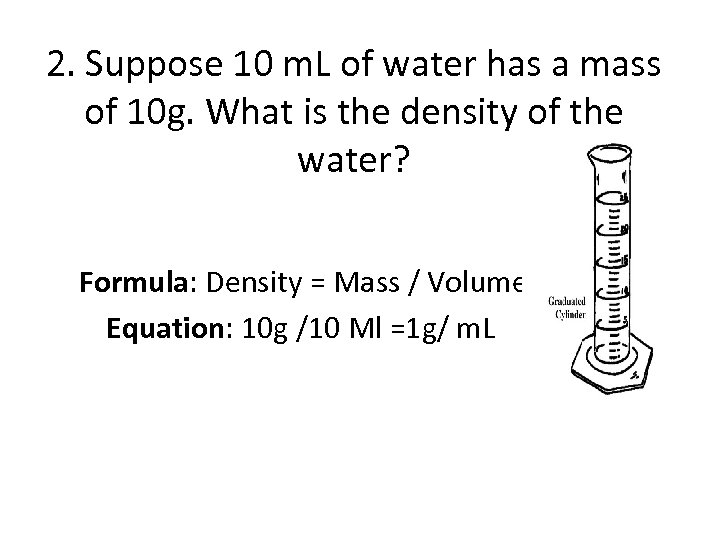2. Suppose 10 m. L of water has a mass of 10 g. What is the density of the water? Formula: Density = Mass / Volume Equation: 10 g /10 Ml =1 g/ m. L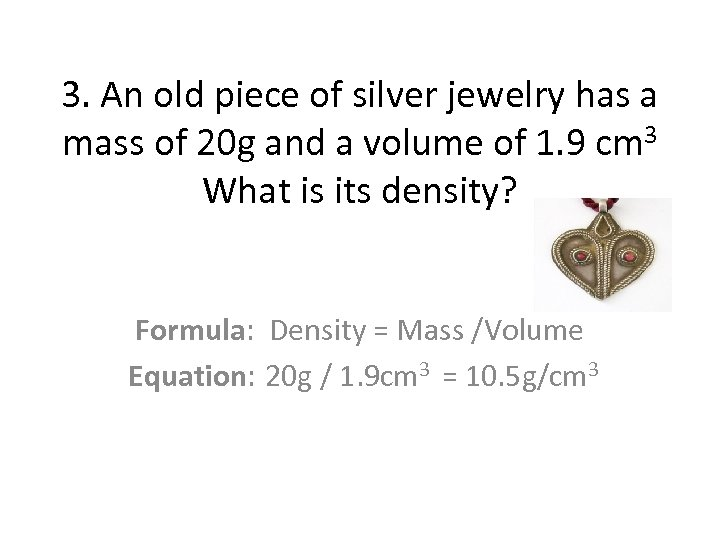3. An old piece of silver jewelry has a mass of 20 g and a volume of 1. 9 cm 3 What is its density? Formula: Density = Mass /Volume Equation: 20 g / 1. 9 cm 3 = 10. 5 g/cm 3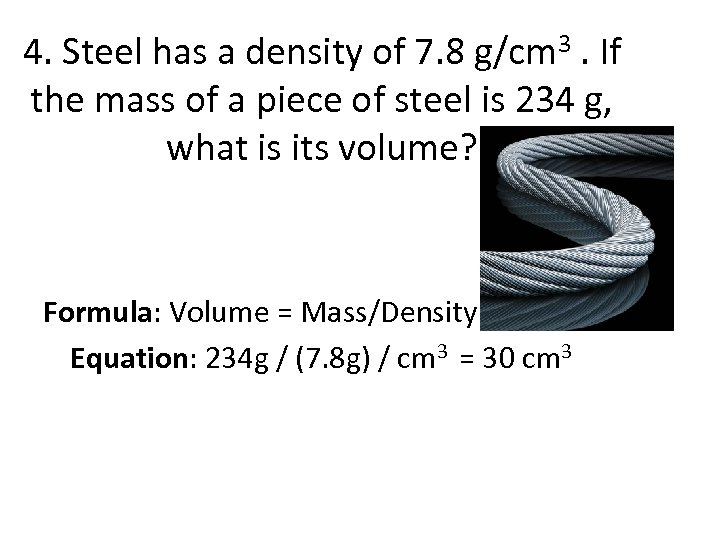4. Steel has a density of 7. 8 g/cm 3. If the mass of a piece of steel is 234 g, what is its volume? Formula: Volume = Mass/Density Equation: 234 g / (7. 8 g) / cm 3 = 30 cm 3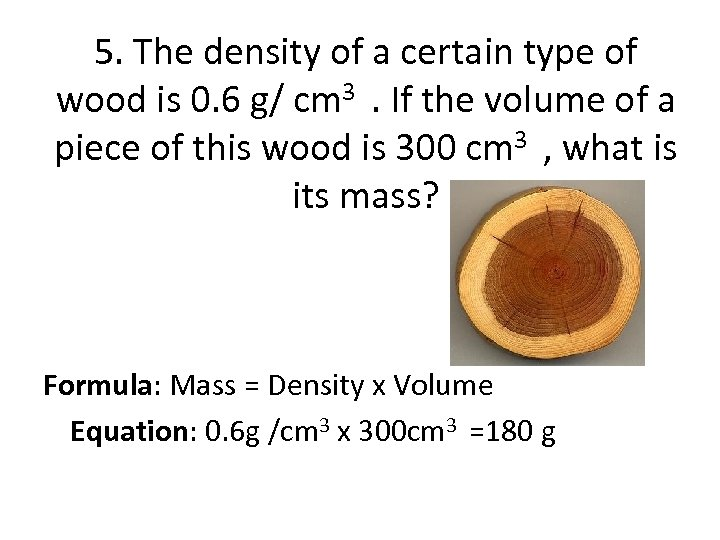5. The density of a certain type of wood is 0. 6 g/ cm 3 . If the volume of a piece of this wood is 300 cm 3 , what is its mass? Formula: Mass = Density x Volume Equation: 0. 6 g /cm 3 x 300 cm 3 =180 g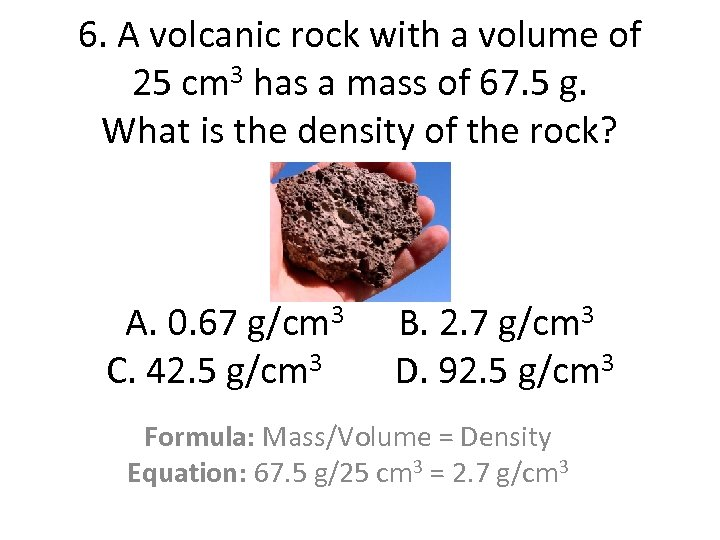6. A volcanic rock with a volume of 25 cm 3 has a mass of 67. 5 g. What is the density of the rock? A. 0. 67 g/cm 3 B. 2. 7 g/cm 3 C. 42. 5 g/cm 3 D. 92. 5 g/cm 3 Formula: Mass/Volume = Density Equation: 67. 5 g/25 cm 3 = 2. 7 g/cm 3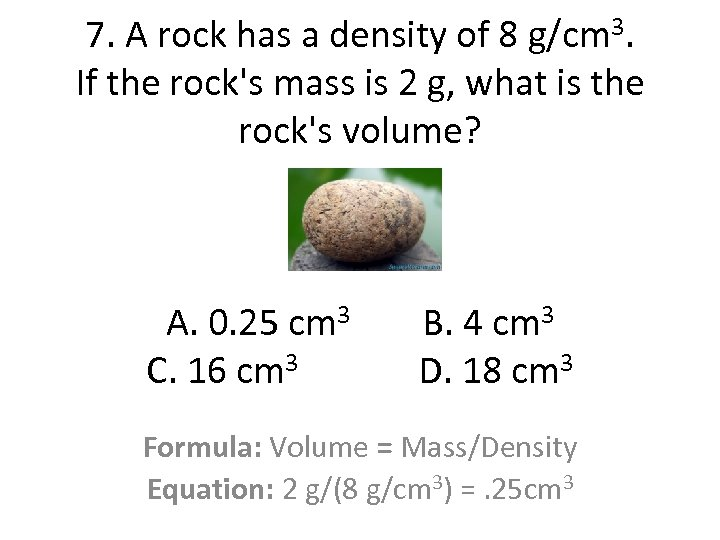7. A rock has a density of 8 g/cm 3. If the rock's mass is 2 g, what is the rock's volume? A. 0. 25 cm 3 B. 4 cm 3 C. 16 cm 3 D. 18 cm 3 Formula: Volume = Mass/Density Equation: 2 g/(8 g/cm 3) =. 25 cm 3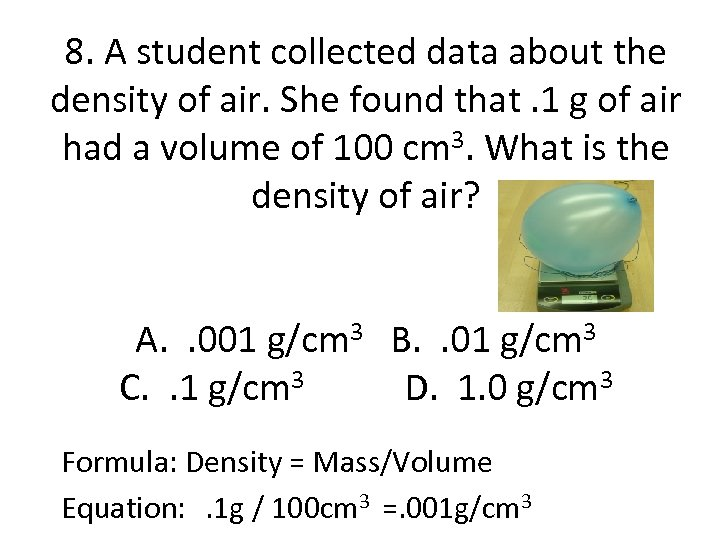8. A student collected data about the density of air. She found that. 1 g of air had a volume of 100 cm 3. What is the density of air? A. . 001 g/cm 3 B. . 01 g/cm 3 C. . 1 g/cm 3 D. 1. 0 g/cm 3 Formula: Density = Mass/Volume Equation: . 1 g / 100 cm 3 =. 001 g/cm 3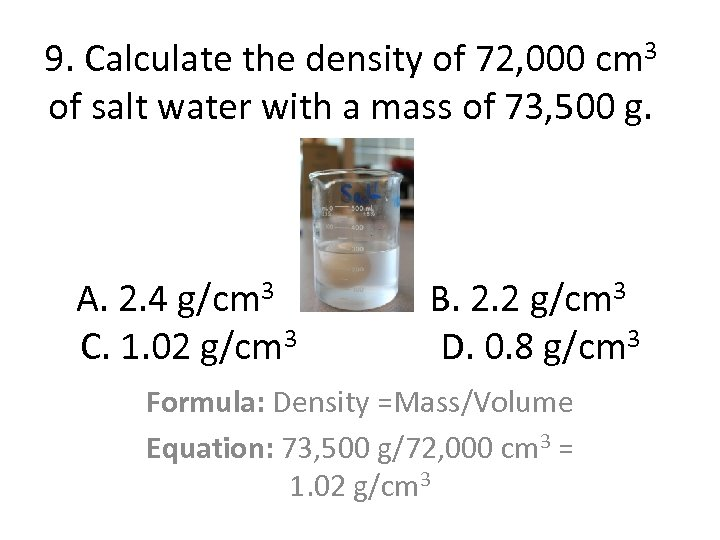9. Calculate the density of 72, 000 cm 3 of salt water with a mass of 73, 500 g. A. 2. 4 g/cm 3 B. 2. 2 g/cm 3 C. 1. 02 g/cm 3 D. 0. 8 g/cm 3 Formula: Density =Mass/Volume Equation: 73, 500 g/72, 000 cm 3 = 1. 02 g/cm 3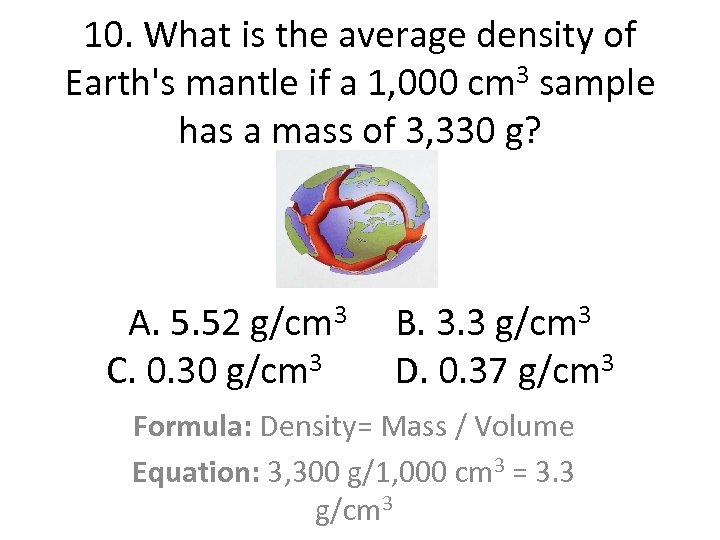10. What is the average density of Earth's mantle if a 1, 000 cm 3 sample has a mass of 3, 330 g? A. 5. 52 g/cm 3 B. 3. 3 g/cm 3 C. 0. 30 g/cm 3 D. 0. 37 g/cm 3 Formula: Density= Mass / Volume Equation: 3, 300 g/1, 000 cm 3 = 3. 3 g/cm 3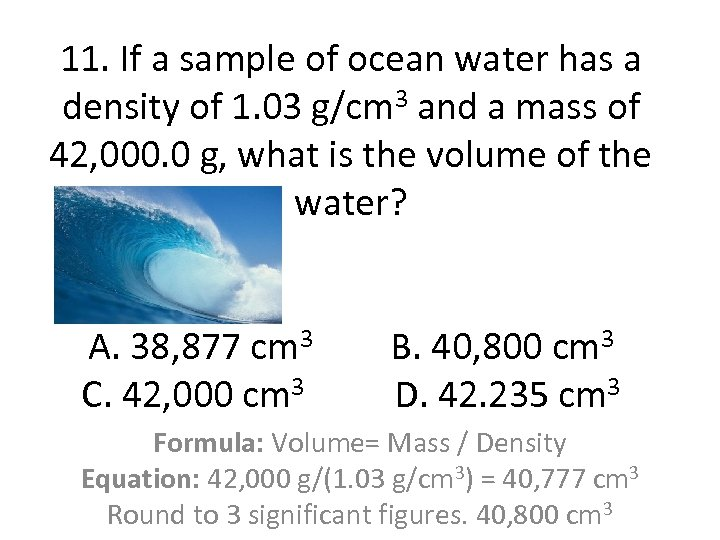11. If a sample of ocean water has a density of 1. 03 g/cm 3 and a mass of 42, 000. 0 g, what is the volume of the water? A. 38, 877 cm 3 B. 40, 800 cm 3 C. 42, 000 cm 3 D. 42. 235 cm 3 Formula: Volume= Mass / Density Equation: 42, 000 g/(1. 03 g/cm 3) = 40, 777 cm 3 Round to 3 significant figures. 40, 800 cm 3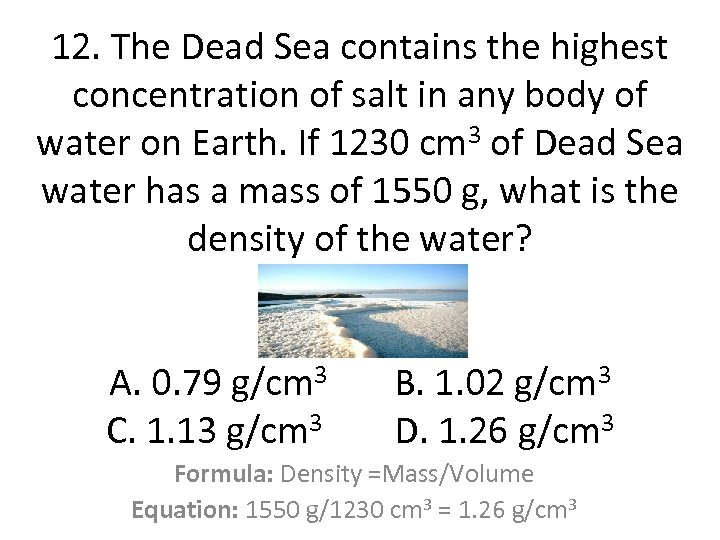12. The Dead Sea contains the highest concentration of salt in any body of water on Earth. If 1230 cm 3 of Dead Sea water has a mass of 1550 g, what is the density of the water? A. 0. 79 g/cm 3 B. 1. 02 g/cm 3 C. 1. 13 g/cm 3 D. 1. 26 g/cm 3 Formula: Density =Mass/Volume Equation: 1550 g/1230 cm 3 = 1. 26 g/cm 3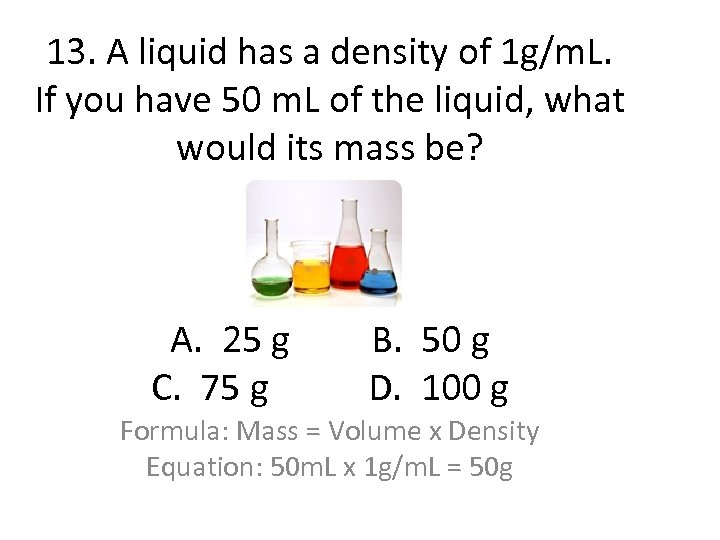13. A liquid has a density of 1 g/m. L. If you have 50 m. L of the liquid, what would its mass be? A. 25 g B. 50 g C. 75 g D. 100 g Formula: Mass = Volume x Density Equation: 50 m. L x 1 g/m. L = 50 g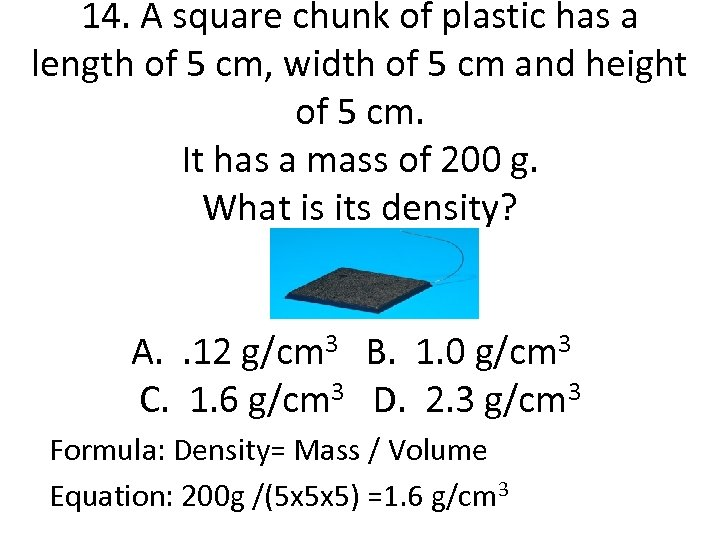14. A square chunk of plastic has a length of 5 cm, width of 5 cm and height of 5 cm. It has a mass of 200 g. What is its density? A. . 12 g/cm 3 B. 1. 0 g/cm 3 C. 1. 6 g/cm 3 D. 2. 3 g/cm 3 Formula: Density= Mass / Volume Equation: 200 g /(5 x 5 x 5) =1. 6 g/cm 3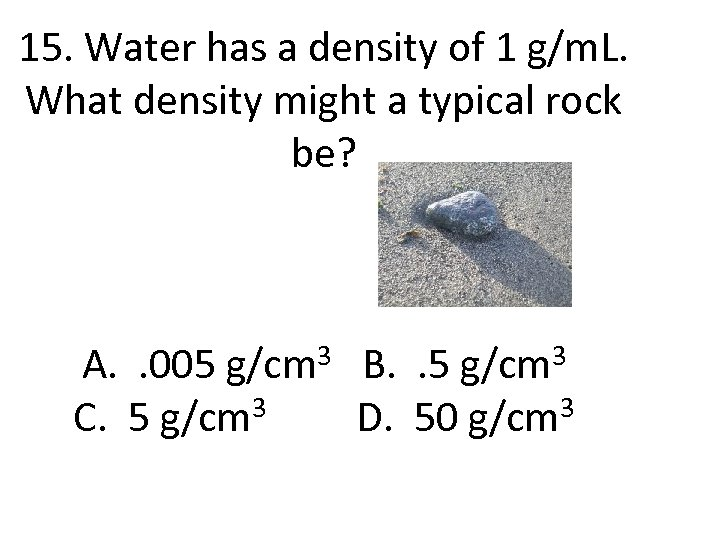15. Water has a density of 1 g/m. L. What density might a typical rock be? A. . 005 g/cm 3 B. . 5 g/cm 3 C. 5 g/cm 3 D. 50 g/cm 3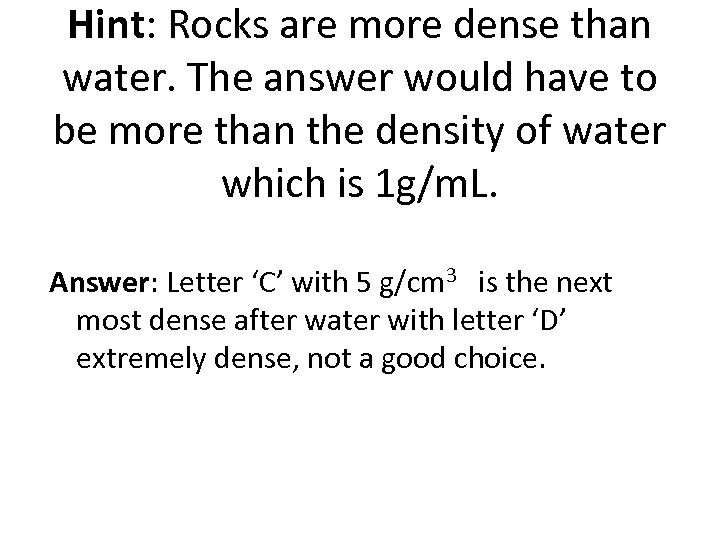Hint: Rocks are more dense than water. The answer would have to be more than the density of water which is 1 g/m. L. Answer: Letter ‘C’ with 5 g/cm 3 is the next most dense after water with letter ‘D’ extremely dense, not a good choice.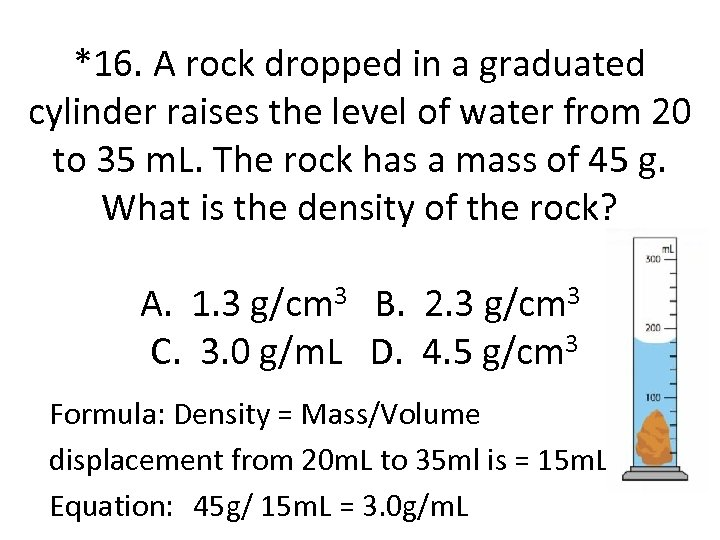*16. A rock dropped in a graduated cylinder raises the level of water from 20 to 35 m. L. The rock has a mass of 45 g. What is the density of the rock? A. 1. 3 g/cm 3 B. 2. 3 g/cm 3 C. 3. 0 g/m. L D. 4. 5 g/cm 3 Formula: Density = Mass/Volume displacement from 20 m. L to 35 ml is = 15 m. L Equation: 45 g/ 15 m. L = 3. 0 g/m. L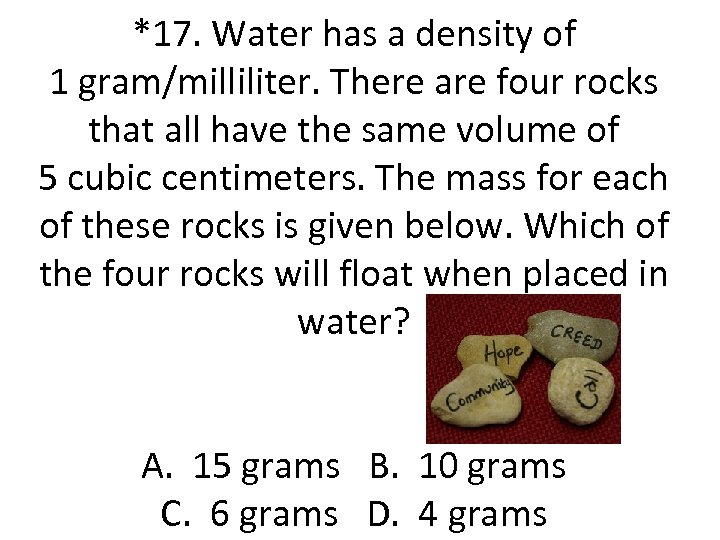*17. Water has a density of 1 gram/milliliter. There are four rocks that all have the same volume of 5 cubic centimeters. The mass for each of these rocks is given below. Which of the four rocks will float when placed in water? A. 15 grams B. 10 grams C. 6 grams D. 4 grams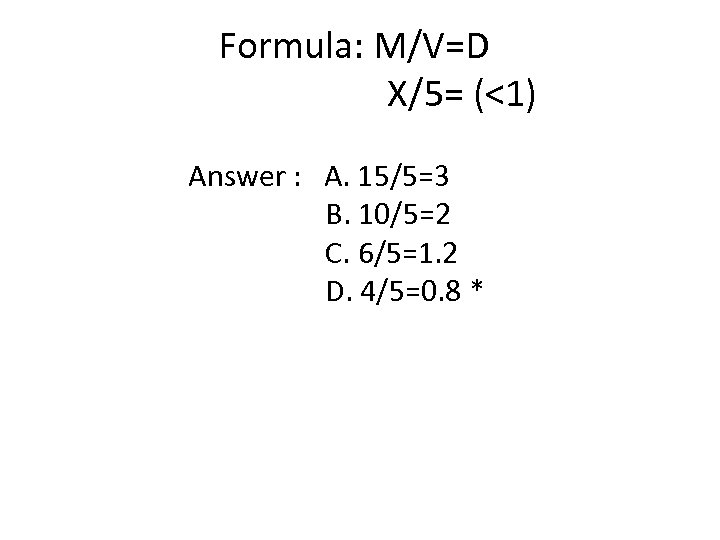Formula: M/V=D X/5= (<1) Answer : A. 15/5=3 B. 10/5=2 C. 6/5=1. 2 D. 4/5=0. 8 *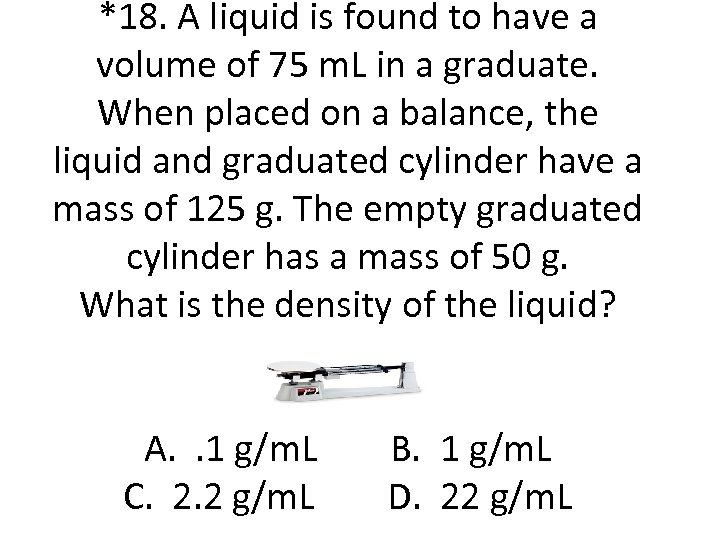*18. A liquid is found to have a volume of 75 m. L in a graduate. When placed on a balance, the liquid and graduated cylinder have a mass of 125 g. The empty graduated cylinder has a mass of 50 g. What is the density of the liquid? A. . 1 g/m. L B. 1 g/m. L C. 2. 2 g/m. L D. 22 g/m. L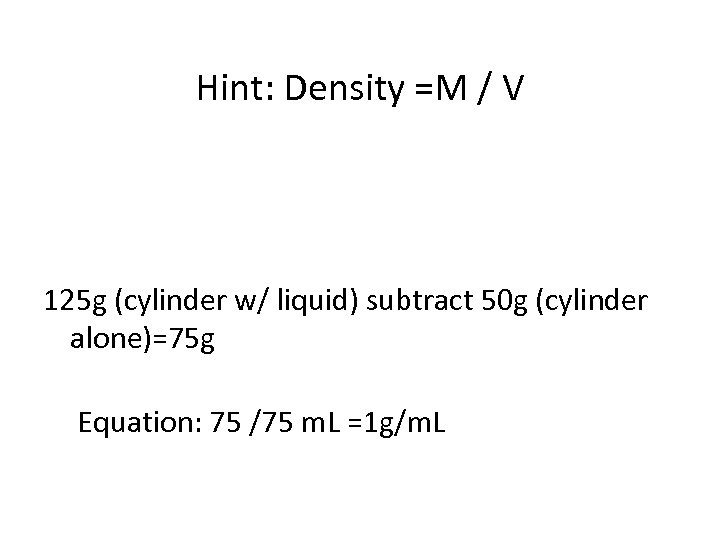Hint: Density =M / V 125 g (cylinder w/ liquid) subtract 50 g (cylinder alone)=75 g Equation: 75 /75 m. L =1 g/m. L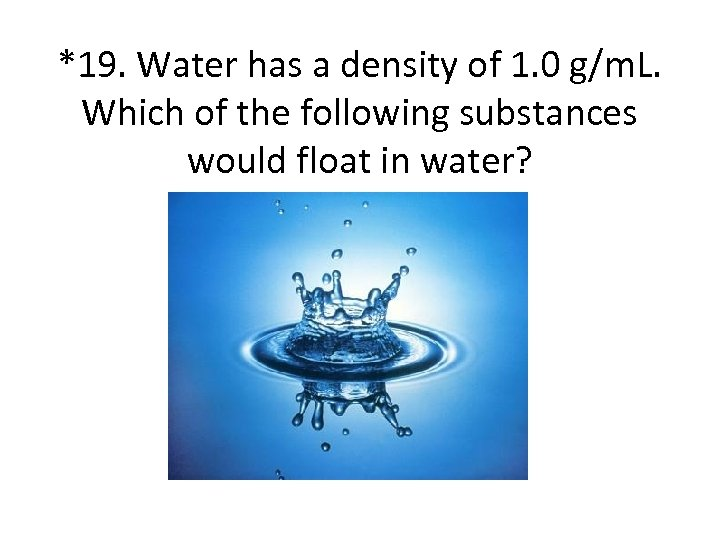*19. Water has a density of 1. 0 g/m. L. Which of the following substances would float in water?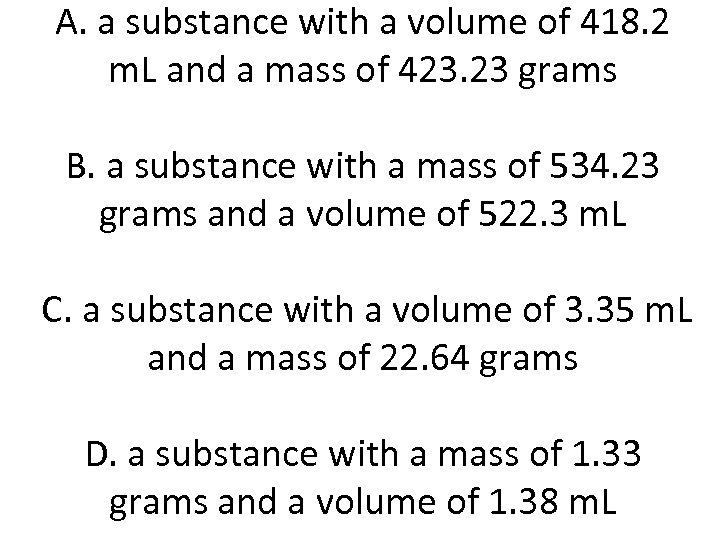A. a substance with a volume of 418. 2 m. L and a mass of 423. 23 grams B. a substance with a mass of 534. 23 grams and a volume of 522. 3 m. L C. a substance with a volume of 3. 35 m. L and a mass of 22. 64 grams D. a substance with a mass of 1. 33 grams and a volume of 1. 38 m. L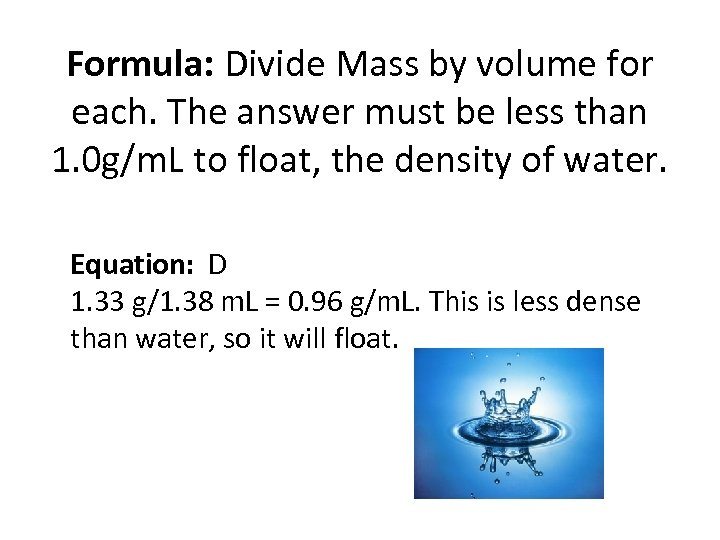Formula: Divide Mass by volume for each. The answer must be less than 1. 0 g/m. L to float, the density of water. Equation: D 1. 33 g/1. 38 m. L = 0. 96 g/m. L. This is less dense than water, so it will float.